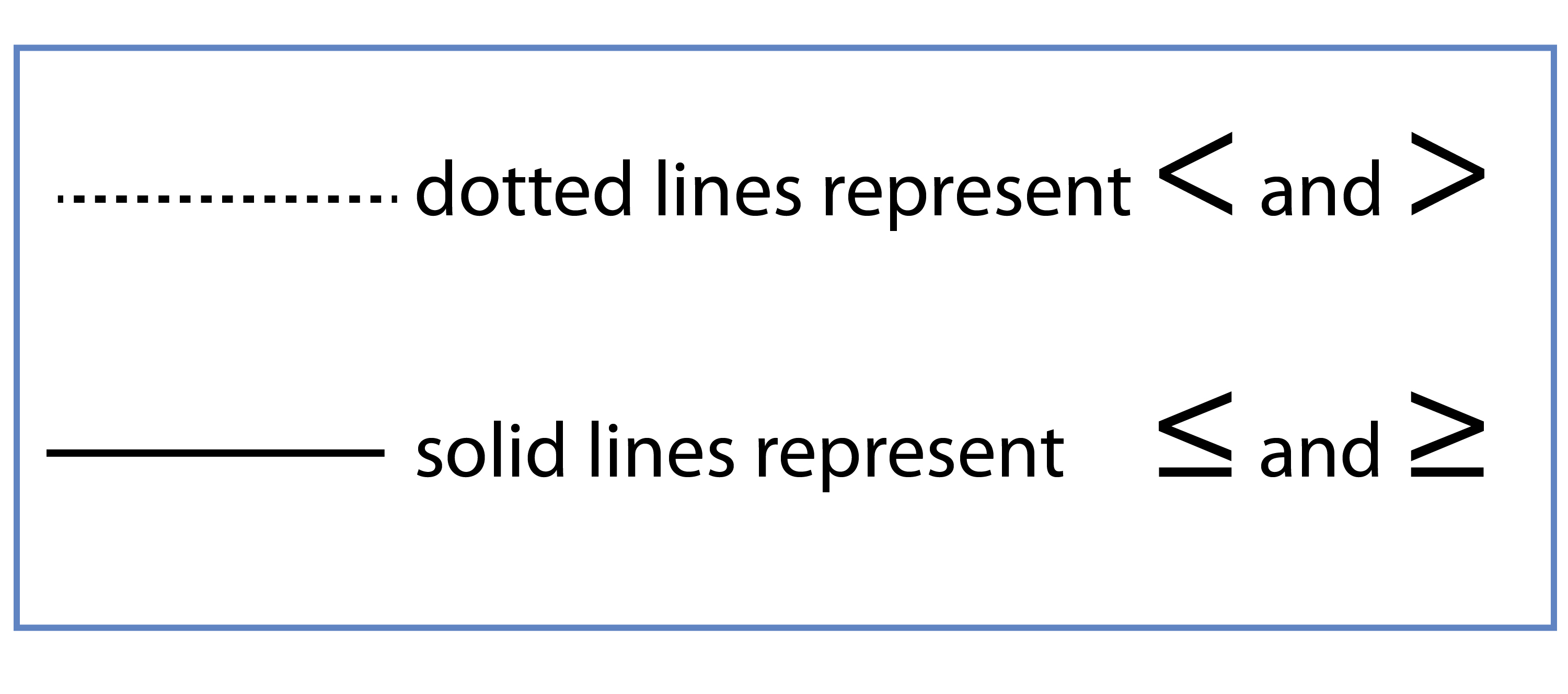# Inequalities and graphs

Inequalities can be represented on a graph and to do that you only need to know two things:Pick a point NOT on the line
If it satisfies the inequality SHADE IT
If it does not satisfy the inequality
(so it must be the other side)

NOTE 1:

We are assuming that when we say “SHADE IT” that that is what the question has asked us to do.

NOTE 2:

In order to draw an inequality graph:

You must understand our section on formula for a straight line.

That is:

1.  Always make y  the subject of the formula

2.  Formula of a straight line is y=mx+c

3.  Plot x and y  guessing values of x